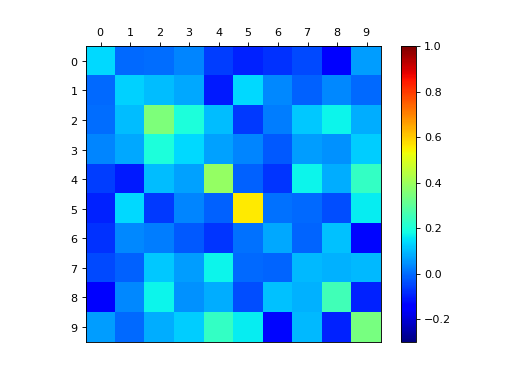# Definition¶

A finite point process $$\mathcal{X}$$ on $$[N] \triangleq \{1,\dots,N\}$$ can be understood as a random subset. It is defined either via its:

• inclusion probabilities (also called correlation functions)

$\mathbb{P}[S\subset \mathcal{X}], \text{ for } S\subset [N],$
• likelihood

$\mathbb{P}[\mathcal{X}=S], \text{ for } S\subset [N].$

Hint

The determinantal feature of DPPs stems from the fact that such inclusion, resp. marginal probabilities are given by the principal minors of the corresponding correlation kernel $$\mathbf{K}$$ (resp. likelihood kernel $$\mathbf{L}$$).

## Inclusion probabilities¶

We say that $$\mathcal{X} \sim \operatorname{DPP}(\mathbf{K})$$ with correlation kernel a complex matrix $$\mathbf{K}$$ if

(1)$\mathbb{P}[S\subset \mathcal{X}] = \det \mathbf{K}_S, \quad \forall S\subset [N],$

where $$\mathbf{K}_S = [\mathbf{K}_{ij}]_{i,j\in S}$$ i.e. the square submatrix of $$\mathbf{K}$$ obtained by keeping only rows and columns indexed by $$S$$.

## Likelihood¶

We say that $$\mathcal{X} \sim \operatorname{DPP}(\mathbf{L})$$ with likelihood kernel a complex matrix $$\mathbf{L}$$ if

(2)$\mathbb{P}[\mathcal{X}=S] = \frac{\det \mathbf{L}_S}{\det [I+\mathbf{L}]}, \quad \forall S\subset [N].$

## Existence¶

Some common sufficient conditions to guarantee existence are:

(3)$\mathbf{K} = \mathbf{K}^{\dagger} \quad \text{and} \quad 0_N \preceq \mathbf{K} \preceq I_N,$
(4)$\mathbf{L} = \mathbf{L}^{\dagger} \quad \text{and} \quad \mathbf{L} \succeq 0_N,$

where the dagger $$\dagger$$ symbol means conjugate transpose.

Note

In the following, unless otherwise specified:

• we work under the sufficient conditions (3) and (3),

• $$\left(\lambda_{1}, \dots, \lambda_{N} \right)$$ denote the eigenvalues of $$\mathbf{K}$$,

• $$\left(\gamma_{1}, \dots, \gamma_{N} \right)$$ denote the eigenvalues of $$\mathbf{L}$$.

# from numpy import sqrt
from numpy.random import rand, randn
from scipy.linalg import qr
from dppy.finite_dpps import FiniteDPP

r, N = 4, 10
e_vecs, _ = qr(randn(N, r), mode='economic')

# Inclusion K
e_vals_K = rand(r)  # in [0, 1]
dpp_K = FiniteDPP('correlation', **{'K_eig_dec': (e_vals_K, e_vecs)})
# or
# K = (e_vecs * e_vals_K).dot(e_vecs.T)
# dpp_K = FiniteDPP('correlation', **{'K': K})
dpp_K.plot_kernel()

# Marginal L
e_vals_L = e_vals_K / (1.0 - e_vals_K)
dpp_L = FiniteDPP('likelihood', **{'L_eig_dec': (e_vals_L, e_vecs)})
# or
# L = (e_vecs * e_vals_L).dot(e_vecs.T)
# dpp_L = FiniteDPP('likelihood', **{'L': K})
# Phi = (e_vecs * sqrt(e_vals_L)).T
# dpp_L = FiniteDPP('likelihood', **{'L_gram_factor': Phi})  # L = Phi.T Phi
dpp_L.plot_kernel()Fig. 1 (png, hires.png, pdf) Correlation $$\mathbf{K}$$ kernelFig. 2 (png, hires.png, pdf) Correlation $$\mathbf{K}$$ kernel

## Projection DPPs¶

Important

$$\operatorname{DPP}(\mathbf{K})$$ defined by an orthogonal projection correlation kernel $$\mathbf{K}$$ are called projection DPPs.

Recall that orthogonal projection matrices are notably characterized by

1. $$\mathbf{K}^2=\mathbf{K}$$ and $$\mathbf{K}^{\dagger}=\mathbf{K}$$,

2. or equivalently by $$\mathbf{K}=U U^{\dagger}$$ with $$U^{\dagger} U=I_r$$ where $$r=\operatorname{rank}(\mathbf{K})$$.

They are indeed valid kernels since they meet the above sufficient conditions: they are Hermitian with eigenvalues $$0$$ or $$1$$.

from numpy import ones
from numpy.random import randn
from scipy.linalg import qr
from dppy.finite_dpps import FiniteDPP

r, N = 4, 10

eig_vals = ones(r)
A = randn(r, N)
eig_vecs, _ = qr(A.T, mode='economic')

proj_DPP = FiniteDPP('correlation', projection=True,
**{'K_eig_dec': (eig_vals, eig_vecs)})
# or
# proj_DPP = FiniteDPP('correlation', projection=True, **{'A_zono': A})
# K = eig_vecs.dot(eig_vecs.T)
# proj_DPP = FiniteDPP('correlation', projection=True, **{'K': K})


## k-DPPs¶

A $$k\!\operatorname{-DPP}$$ can be defined as $$\operatorname{DPP(\mathbf{L})}$$ (2) conditioned to a fixed sample size $$|\mathcal{X}|=k$$, we denote it $$k\!\operatorname{-DPP}(\mathbf{L})$$.

It is naturally defined through its joint probabilities

(5)$\mathbb{P}_{k\!\operatorname{-DPP}}[\mathcal{X}=S] = \frac{1}{e_k(L)} \det \mathbf{L}_S ~ 1_{|S|=k},$

where the normalizing constant $$e_k(L)$$ corresponds to the elementary symmetric polynomial of order $$k$$ evaluated in the eigenvalues of $$\mathbf{L}$$,

$\begin{split}e_k(\mathbf{L}) \triangleq e_k(\gamma_1, \dots, \gamma_N) = \sum_{\substack{S \subset [N]\\|S|=k}} \prod_{s\in S} \gamma_{s} = \sum_{\substack{S \subset [N]\\|S|=k}} \det L_S.\end{split}$

Note

Obviously, one must take $$k \leq \operatorname{rank}(L)$$ otherwise $$\det \mathbf{L}_S = 0$$ for $$|S| = k > \operatorname{rank}(L)$$.

Warning

k-DPPs are not DPPs in general. Viewed as a $$\operatorname{DPP}$$ conditioned to a fixed sample size $$|\mathcal{X}|=k$$, the only case where they coincide is when the original DPP is a projection $$\operatorname{DPP}(\mathbf{K})$$, and $$k=\operatorname{rank}(\mathbf{K})$$, see (13).